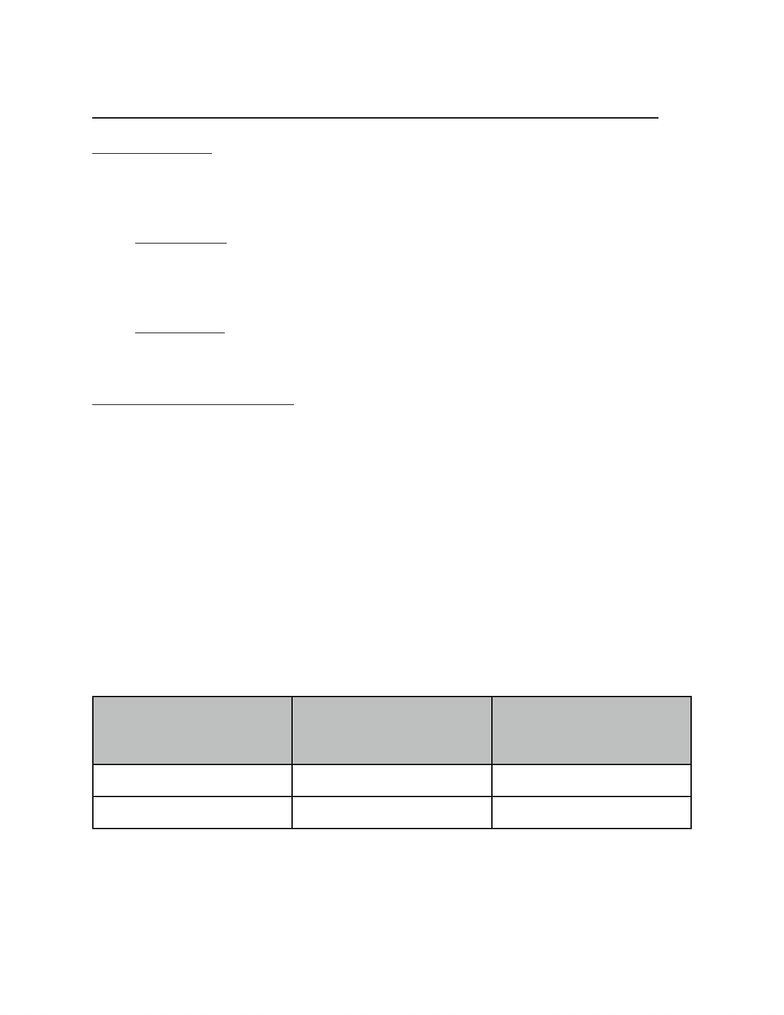Study Guides (400,000)
CA (160,000)
Brock U (1,000)
ECON (60)
Midterm

# ECON 1P91 Study Guide - Midterm Guide: Inferior Good, Normal Good, Price Ceiling

Department
Economics
Course Code
ECON 1P91
Professor
Professor Cottrel
Study Guide
Midterm

This preview shows pages 1-2. to view the full 6 pages of the document.Class #5! Chapter 4 Cont. !Sept. 29, 2011
Income Elasticity:
- Responsiveness of Demand to a change in Income
nI = %DELTA Q^d / %DELTA Income
nI > 0 Normal Good
- nI > 1 Income Elastic
- nI =< 1 Income Inelastic
nI < 0 Inferior Good
- As income increases
- Quantity demanded decreases
Normal Good (Luxury Goods)
nI > 1 Income Elastic [ positive ]
- As income increases
- Quantity demanded increases by a greater % than income increases
nI =< 1 Income Inelastic [ positive ]
- As income increases
- Quantity demanded increases by a smaller % than income increases
Problem:
-1 good - ﬂowers
-Income increases from \$40,000 to \$50,000
-Find the income elasticity of demand
-Normal Good or Inferior good?
Explain.
Price
Flowers
Q^d
Flowers
Income = \$40,000
Q6d
Flowers
Income = \$50,000
\$1.00
200
250
\$2.00
160
220
*** Price of ﬂowers constant
nI != %DELTA Q^d / %DELTA I!!!!P = \$1
nI != ((250-200) / ((250 + 200) / 2)) / ((50,000-40,000) / ((50,000+40,000) / 2))
nl != (50/25) / (10,000/45,000)

Only pages 1-2 are available for preview. Some parts have been intentionally blurred.nl!= 0.222 / 0.222
nl!= + 1
nI > 0 Positive Normal Good
As income increases, the demand for ﬂowers - a normal good - also increases.
[ nI =< 1 Income Inelastic ]
-As income increases, Quantity of ﬂowers demanded increases by a smaller % than
income increases
Elasticity of Supply:
- Degree to which Quantity Supplied responds to changes in Price (Ceteris Paribus)
- Refer to diagram 1 & 2
nS = %DELTA Q^s / %DELTA P
nS < 1 Inelastic
%DELTA Q^s < %DELTA P
nS > 1 Elastic
%DELTA Q^s > %DELTA P
Determinants of Supply:
Resource Substitution:
-Use unique or rare resources in production nS = 0
-perfectly inelastic [ or, highly inelastic ]
- Refer to diagram 3
-[ No %DELTA Q^s ] > %DELTA P
-Almost perfect substitute nS = Inﬁnite
-perfectly elastic [ or, highly elastic ]
- Refer to diagram 4
-%DELTA Q^s > [ No %DELTA P ] (given P)
- Unit Elastic nS = 1
- Through Origin
- Refer to diagram 5
-%DELTA Q^s = %DELTA P
Time Frame:
- Short Run
- Inelastic nS < 1
-Long Run
- Elastic nS > 1
- More time to change process
-Refer to diagram 6